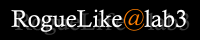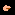# @ The NetHack CodeOriginal HTML version (http://blues.helsinki.fi/%7Evviitane/nhcode.html)

\$BLu \$BF|K\8lLu\$K4X\$9\$k(B \$B%3%a%s%H(B \$B\$*\$h\$S(B \$B%P%0Js9p(B \$B\$O(B, RogueLike@lab3 \$B4IM} \$B\$^\$G(B \$B\$*4j\$\$\$7\$^\$9!#(B

\$BJT=8 Any comments you have regarding this are best discussed in the newsgroup rec.games.roguelike.nethack, or if they are related to the html version, you can mail the editor directly.

## The NetHack Code 1.1

Ed Chang \$B\$,;W\$\$\$D\$-(B Dan Shiovitz \$B\$,;O\$a\$?%"%\$%G%#%"\$K4p\$E\$-(B, Raisse the Thaumaturge (irina@rempt.xs4all.nl, in real life: Irina Rempt-Drijfhout) \$B\$K\$h\$C\$F%a%s%F%J%s%9\$5\$l\$F\$\$\$^\$9!#(B

### \$B%t%!!<%8%g%s\$K\$D\$\$\$F!'(B

\$B\$3\$N%t%!!<%8%g%s\$O(B, \$B%P%0%U%#%/%9\$N\$_\$rL\E*\$H\$7\$?\$b\$N\$G\$9!#(B \$B2~NI\$O(B version 2 \$B\$G0Y\$5\$l\$kM=Dj\$G\$9!#(B

\$BM=Dj\$5\$l\$F\$\$\$k2~NI!'(B \$B:b;:(B, \$B%W%l%\$\$NAa\$5(B, \$B0!

\$B\$3\$N%t%!!<%8%g%s\$K\$*\$1\$k2~NI!'(B Viljo Viitanen \$B\$5\$s\$NL>A0\$,=\$@5\$5\$l\$?(B (i\$B\$,\$U\$?\$D(B, by Crom \$B\$5\$s\$K\$h\$k(B)\$B!((B \$B\$\$\$/\$D\$+\$N4V0c\$\$\$,=\$@5\$5\$l\$?!((B So \$B\$H(B C \$B\$r%^%\$%J!<%A%'%s%8(B, X \$B\$r%a%8%c!<%A%'%s%8(B, \$B\$3\$l\$i\$O(B Viljo Viitanen \$B\$5\$s(B (vviitane@cc.helsinki.fi) \$B\$H(B Daniel A. Davis \$B\$5\$s(B (davis@cc.helsinki.fi) \$B\$K\$h\$k!((B \$B\$\$\$/\$D\$+\$N%9%Z%k%_%9\$,=\$@5\$5\$l\$?(B (\$B\$G\$bB?J,\$^\$@;D\$C\$F\$k(B :-()\$B!#(B